Next: Poincaré Invariants Up: Charged Particle Motion Previous: Magnetic Drifts

# Invariance of Magnetic Moment

Let us now demonstrate that the magnetic moment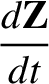is indeed a constant of the motion, at least to lowest order. The scalar product of the equation of motion (59) with the velocity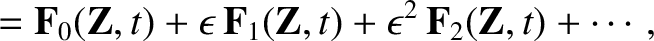yields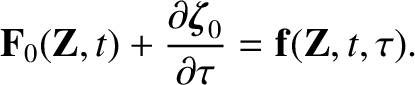(95)

This equation governs the evolution of the particle energy during its motion. Let us make the substitution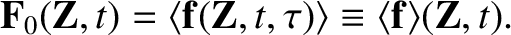, as before, and then average the above equation over gyrophase. To lowest order, we obtain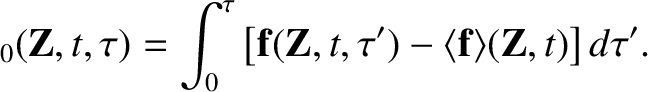(96)

Here, use has been made of the result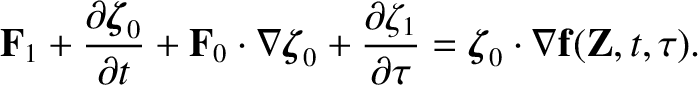(97)

which is valid for any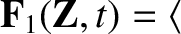. The final term on the right-hand side of Eq. (96) can be written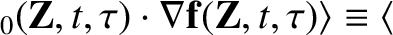(98)

Thus, Eq. (96) reduces to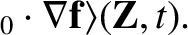(99)

Here,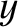is the guiding centre velocity, evaluated to first order, and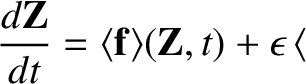(100)

is the kinetic energy of the particle. Evidently, the kinetic energy can change in one of two ways. Either by motion of the guiding centre along the direction of the electric field, or by the acceleration of the gyration due to the electromotive force generated around the Larmor orbit by a changing magnetic field.

Equations (70), (85), and (86) can be used to eliminate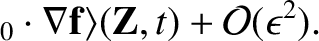and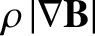from Eq. (99). The final result is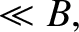(101)

Thus, the magnetic moment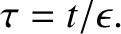is a constant of the motion to lowest order. Kruskalhas shown that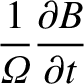is the lowest order approximation to a quantity which is a constant of the motion to all orders in the perturbation expansion. Such a quantity is called an adiabatic invariant.Next: Poincaré Invariants Up: Charged Particle Motion Previous: Magnetic Drifts
Richard Fitzpatrick 2011-03-31